ISSAC 97 - Meijer G Function Representations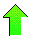Contents News View

 Contiguity Relations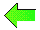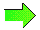Operator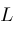is a polynomial in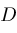but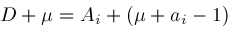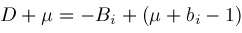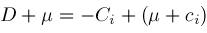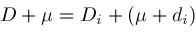socan also be expressed as a polynomial in terms of shift operators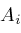,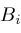,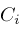, and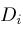converting the differential equation for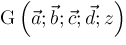into a difference equation among contiguous instances of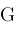which we call a contiguity relation.

Let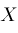stand for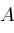,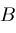,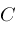, orand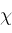stand for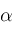,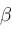,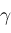, or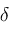respectively.  If we expressas a polynomial in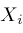, then we get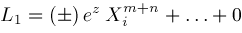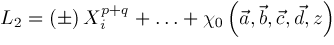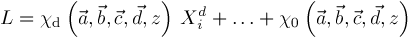where the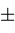signs depend on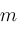,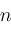,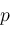,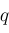and whetheris,,,and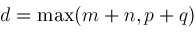These results let us define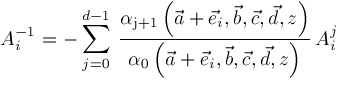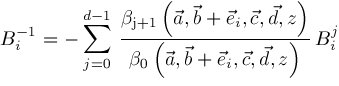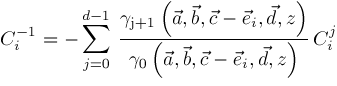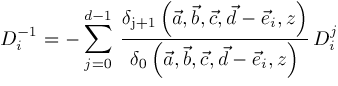The coefficients of these polynomials in,,,
andare defined when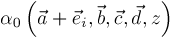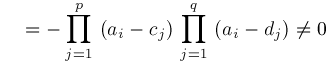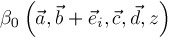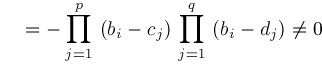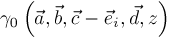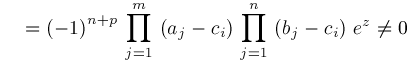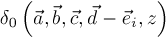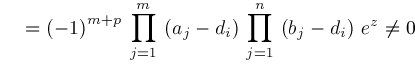Operators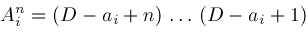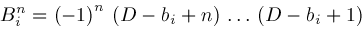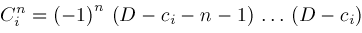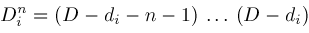are defined for all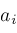,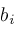,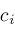, and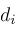©2004-2021 Planet Quantum Kelly Roach